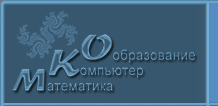English
 ! Вопросы и ответы

# Тезисы

## Semi-analytical methods for simulation of diffusion-influenced reactions on active particles

Traytak S.D.

Semenov Institute of Chemical Physics RAS, 4 Kosygina St., 117977 Moscow, Russia, Tel.: (495) 939-7101, E-mail: sergtray@mail.ru

1  стр. (принято к публикации)

Despite some advantages of existing numerical methods for solution of the diffusion equation in arbitrary 3D domains they all bear a common deﬁciency related to the necessity of discretization of either the boundary surfaces, or of the configuration space. Fortunately for simpliﬁed geometries, such as multiple canonical domains (sets of circular and elliptic cylinders, spheres, ellipsoids, etc.) the diffusion problem can be efficiently solved by means of semi-analytical the generalized method of separation of variables (GMSV). Substantially this method is based on application of appropriate addition theorems for the external and internal basic solutions to the diffusion equation. The GMSV is analytical since it is based on solutions in the form of infinite series with respect to basic solutions. On the other hand this method is numerical, since it requires inversion of a large size so called interaction matrix for determining unknown coefficients in the series, to satisfy boundary conditions on multiple canonical surfaces. Finally, the GMSV procedure leads to the Fredholm II kind inﬁnite systems of linear algebraic equations (ISLAE) with respect to the coefficients of the expansions. Note that for the case of compact interaction matrix operator these ISLAE may be easily solved numerically by the reduction method.

With the aid of the GMSV we develop here so called “Lego” principle suggested in our previous studies. Like Lego construction toys, different canonical domains can be assembled and connected in many ways, to construct adequate models for real chemical and biological objects (such as polymers, antibodies molecules, bacteria, viruses etc.). As an important specific application of the “Lego” principle we consider the model for bacterial chemoreception, representing the receptors as small spherical ideally absorbing sinks which cover part of the baсterium spherical surface of an otherwise reflecting one.

We also investigate the binding of diffusing particles into a 3D spherical region randomly filled by spherical absorbing cells. It has been shown that well-known mean-field diffusion equation for the coarse-grained particle concentration may be used only deep inside the above spherical array of cells. In addition, to describe the behavior of the coarse-grained particle concentration in the diffusion layer near the array boundary one should use new diffusion equation with some appropriate matching conditions derived by means of the renormalization group approach. It is worth noting that the diffusive interactions between cells are taken into account by employing the monopole approximation, which proved to be rather good one for the spherical cells. Our theory predicts a penetration length which is much larger than it follows from all known mean-field theories. Among many different applications, we note that obtained results may be immediately used, e.g., to study diffusion-influenced processes in yeast colonies which are growing due to an abundance of glucose.

© 2004 Дизайн Лицея Информационных технологий №1533